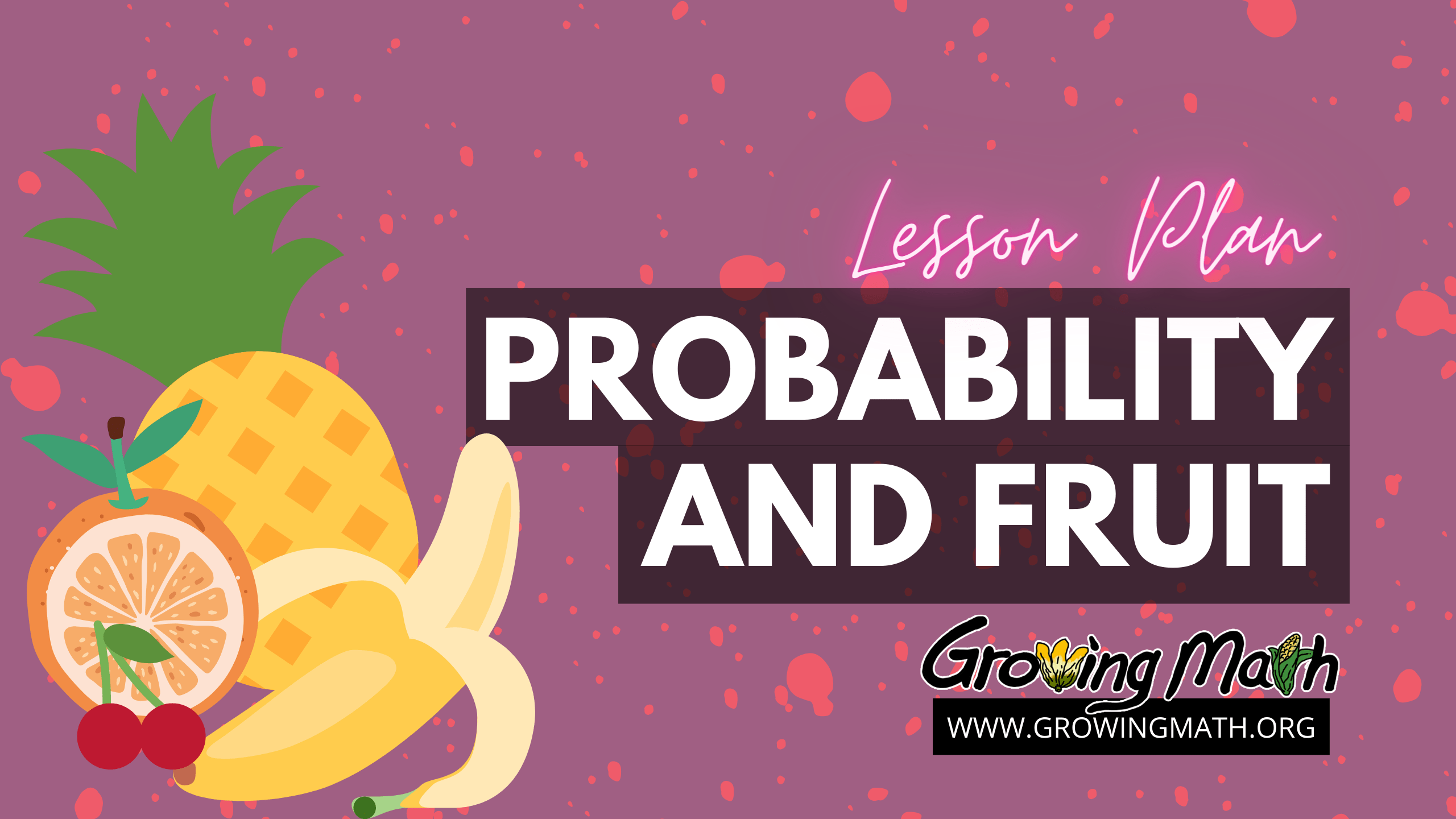Author Archives: Zeb HammondProbability and fruit

📖Standards

CCSS.Math.Content.7.SP.C.5
Understand that the probability of a chance event is a number between 0 and 1 that expresses the likelihood of the event occurring. Larger numbers indicate greater likelihood. A probability near 0 indicates an unlikely event, a probability around 1/2 indicates an event that is neither unlikely nor likely, and a probability near 1 indicates a likely event.

CCSS.Math.Content.7.SP.C.6
Approximate the probability of a chance event by collecting data on the chance process that produces it and observing its long-run relative frequency, and predict the approximate relative frequency given the probability.

⏰Time

30-45 minutes, depending on whether the final individual activity is performed in class or outside of class.

📲Technology Required

A projector or smart board is required to show the Google slides presentation in class. It can also be shown using any web meeting software for remote learning. The random fruit basket generator can be used by students in anything with a browser, including computers, tablets or phones. This activity is optional.

📃Summary

The Google slides presentation begins with definitions of probability, impossibility and certainty. Students are then given an example of a basket with different types of fruits and the probability of each. Students each come to the front of the class and pull a piece of fruit from the basket, writing down the probability of their selecting the type they obtained. The class data is used to create a table and compare the obtained probabilities to actual distribution of fruit in the basket. The lesson closes with students creating their own probability question.

NOTE: This lesson plan requires a basket of fruit. You could use pictures of fruit printed out or drawn on paper instead but using actual fruit from your area might be more fun. If you’d rather, though, we do have a random fruit basket generator to use with this lesson.

📚Lesson Plan

1. Presentation on Probability

The Google slides presentation begins with definitions of probability, impossibility and certainty. Students are then given an example of a basket with different types of fruits and the probability of each. Students each come to the front of the class and pull a piece of fruit from the basket, writing down the probability of their selecting the type they obtained. The class data is used to create a table and compare the obtained probabilities to actual distribution of fruit in the basket. The lesson closes with

Students are then given an example of a basket with different types of fruits and the probability of each.

2. Individual students compute probability

Students each come to the front of the class and pull a piece of fruit from the basket, writing down the probability of their selecting the type they obtained. The class data is used to create a table and compare the obtained probabilities to actual distribution of fruit in the basket. If students are learning at home, we have a random fruit basket generator to use with this lesson. After setting THE SAME NUMBER OF EACH FRUIT for everyone to enter, have each student click on the basket to select a random fruit and see the probability.

3. Group Activity

Complete table included in presentation as a class. Compare obtained probabilities with expected. Discuss that the expected probabilities are for a large number of trials and that it is usual to have the observe probabilities not match up exactly – or even closely – with the actual proportions when the sample size is small.

4. Probing questions

These questions are included in the presentation. Ask the class what the probability is of selecting a kiwi fruit, remembering that there are not any kiwi in the basket. If Annie’s family owns an apple orchard and her basket contains only apples, what is the probability of selecting an apple? If students need a reminder, return to the definitions of probability, impossibility and certainty at the beginning of the lesson.

5. Individual Activity

Students will create their own probability activity using household items. This activity can be performed at home, having family members draw items, or can be performed in the classroom using items in the classroom, such as different colors of pencils, or even pieces of paper with different words or pictures.

Assessment

Individual formative assessment is conducted throughout this activity by having students write the probability of the specific fruit they selected and by their performance on the individual activity creating their own probability sample and recording the results. Students can also write their answers to the in-class questions on probabilities of 0 and 1, then comparing these to the correct answer.

State Standard

Missouri Learning Standards (MLS)●7.DSP.C.5a Understand that the probability of a chance event is a number between 0 and 1 that expresses the likelihood of the event occurring. Larger numbers indicate greater likelihood. A probability near 0 indicates an unlikely event, a probability around 1/2 indicates an event that is neither unlikely nor likely, and a probability near 1 indicates a likely event.

Minnesota Math Standard 6.4.1.2 – Determine the probability of an event using the ratio between the size of the event and the size of the sample space; represent probabilities as percents, fractions and decimals between 0 and 1 inclusive. Understand that probabilities measure likelihood.

Minnesota Math Standard 6.4.1.4 – Calculate experimental probabilities from experiments; represent them as percents, fractions and decimals between 0 and 1 inclusive. Use experimental probabilities to make predictions when actual probabilities are unknown.